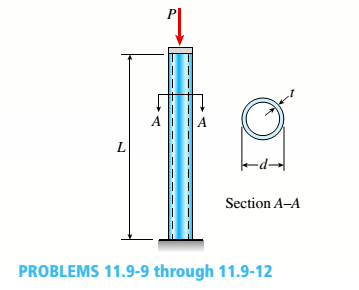# - 11 Determine the maximum permissible length L m a x for a steel pipe column that is fixed at the base and free at the top and must support an axial load P = 40 kips (see figure). The column has an outside diameter d = 4.0 in., wall thickness t = 0.226 in., E = 29,000 ksi, and σ y = 42 ksi.### Mechanics of Materials (MindTap Co...

9th Edition
Barry J. Goodno + 1 other
Publisher: Cengage Learning
ISBN: 9781337093347### Mechanics of Materials (MindTap Co...

9th Edition
Barry J. Goodno + 1 other
Publisher: Cengage Learning
ISBN: 9781337093347
Chapter 11, Problem 11.9.11P
Textbook Problem
1 views

## -11 Determine the maximum permissible length Lmaxfor a steel pipe column that is fixed at the base and free at the top and must support an axial load P = 40 kips (see figure). The column has an outside diameter d = 4.0 in., wall thickness t = 0.226 in., E = 29,000 ksi, and σ y = 42 ksi.To determine

The value of the maximum permissible length Lmax for a steel pipe column.

### Explanation of Solution

Given Information:

The outside diameter of column, do = 4.0 in.

The thickness of the wall of column, t = .226 in.

The allowable load, P = 40 k

The value of the young modulus, E = 29000 ksi

The value of maximum stress, sy = 36 ksi

Concept Used:

For fixed free end, K=2

di=do2tA=π4(do2di2)I=π64(do4di4)r=IA( L r)max=200

Here, r = radius of gyration.

Lc=r 2 π 2 E σ y Lc=critical length of column in ft

i=1,2...4n1i=NA          ifKLir>KLcr&n1i=53+3( K L i r )8( K L c r ) ( K L i r )38 ( K L c r )3ifKLirKLcrn2i=2312          ifKLir>KLcr&n2i=NA          ifKLirKLcr

σallow=σy[1 n 1 i (1 ( K L i r ) 2 2 ( K L c r ) 2 )]ifKLirKLcrσallow=σy[ ( K L c r ) 22 n 2 i ( K L i r ) 2]ifKLir>KLcrPallow=σallow×A

Calculation:

di=4.02(.226)=3.548in.A=π4(42 3.5482)=2.6795in.2I=π64(44 3.5484)=4.7877in.4r= 4.7877 2.6795=1.3367in.( KL r)max=200Lmax=200×1.3367/2=133.67in

### Still sussing out bartleby?

Check out a sample textbook solution.

See a sample solution

#### The Solution to Your Study Problems

Bartleby provides explanations to thousands of textbook problems written by our experts, many with advanced degrees!

Get Started

Find more solutions based on key concepts
What is a DBMS, and what are its functions?

Database Systems: Design, Implementation, & Management

In Chapter 10, we discussed fluid pressure and the role of water towers in small towns. Use MATLAB to create a ...

Engineering Fundamentals: An Introduction to Engineering (MindTap Course List)

What is a common problem in calculating start and finish times? Provide an example.

Systems Analysis and Design (Shelly Cashman Series) (MindTap Course List)

Primary key a. procedures that keep data current b. item that contains data, as well as the actions that read o...

Enhanced Discovering Computers 2017 (Shelly Cashman Series) (MindTap Course List)

If your motherboard supports ECC DDR3 memory, can you substitute non-ECC DDR3 memory?

A+ Guide to Hardware (Standalone Book) (MindTap Course List)